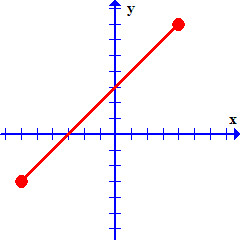# How to Use The Midpoint Formula

Instructions:

Choose an answer and hit 'next'. You will receive your score and answers at the end.

question 1 of 3

### Which of the following graphs shows a line segment?

Create Your Account To Take This Quiz

As a member, you'll also get unlimited access to over 75,000 lessons in math, English, science, history, and more. Plus, get practice tests, quizzes, and personalized coaching to help you succeed.

Try it risk-free for 30 days. Cancel anytime.

### 2. Estimate the coordinates of the midpoint of the line segment below:Create your account to access this entire worksheet
A Premium account gives you access to all lesson, practice exams, quizzes & worksheets
Quizzes, practice exams & worksheets
Certificate of Completion
Create an account to get started

The midpoint formula is an important concept to know when studying line segments and planes, and this quiz/worksheet will help you test your understanding of how to use it. You'll also be tested on some related mathematical terminology.

## Quiz & Worksheet Goals

In these assessments you'll be tested on the identification, definition and application of:

• Line segments
• Endpoints
• The midpoint formula

## Skills Practiced

• Problem solving - use acquired knowledge to solve practice problems involving coordinates and the midpoint formula
• Defining key concepts - ensure that you can accurately define and identify important terms, such as line segment and endpoints
• Knowledge application - use your knowledge to answer questions using the midpoint formula

To master the various subtleties of the midpoint formula, you should read the related lesson. It is called How to Use The Midpoint Formula. The lesson has these objectives:

• Define line segment
• Define midpoint
• Know when to use the midpoint formula
• Understand the function of the midpoint formula
• Understand the midpoint formula through provided examples
Final ExamMath 102: College Mathematics
Status: Not Started
Chapter ExamMath Foundations
Status: Not Started

Support